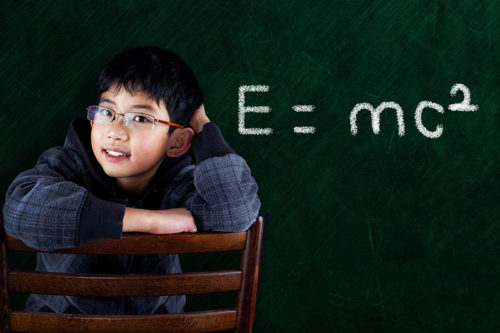# P4-P6 Math Tuition

## Math Tuition For Primary 5 and 6

At Einstein Education Hub, we have crafted out our math tuition to suit our primary 5 and primary 6 students. Our primary 5 math tuition classes are meant to provide our primary 5 students confidence before they move on to primary 6. Most of our primary 5 students who attend our math tuition have developed a strong foundation in their mathematics syllabus as they prepare themselves for the O Levels examination at the end of their primary 6 enrolment.

## PROGRAM COVERAGE

### P4 – P6 MathematicsUpper Primary Math

 NUMBERS WITH LARGE VALUES Identify and recognize the differencebetween hundred thousands and millions. Work out the addition, subtraction, division and multiplications of small and large values fast and accurately FRACTIONS Master the concepts of fraction and relate fractions to values, decimals and percentages. Work out the addition, subtraction, division and multiplication of fraction correctly and draw models to represent fractions accurately RATIOS Understand the difference between ratios and compare the different ratio using the correct techniques. Link up the ratios with fractions and percentages and draw model to represent ratio correctly
 PERCENTAGE Understand the difference between percentage of different quantities and convert to the actual percentage of the common quantity Convert percentage to decimal and fraction and draw model to represent percentage accurately SHORT ANSWER QUESTIONS Show all the steps and workings clearly. Do step by step and label each step what it represents PROBLEM SUMS Analyze the problem sums wish deep understanding using models and logical reasonings. Do step by step, clearly labelling each step with what it represents and derive the answers correctly after reviewing the workings and explanations thoroughly